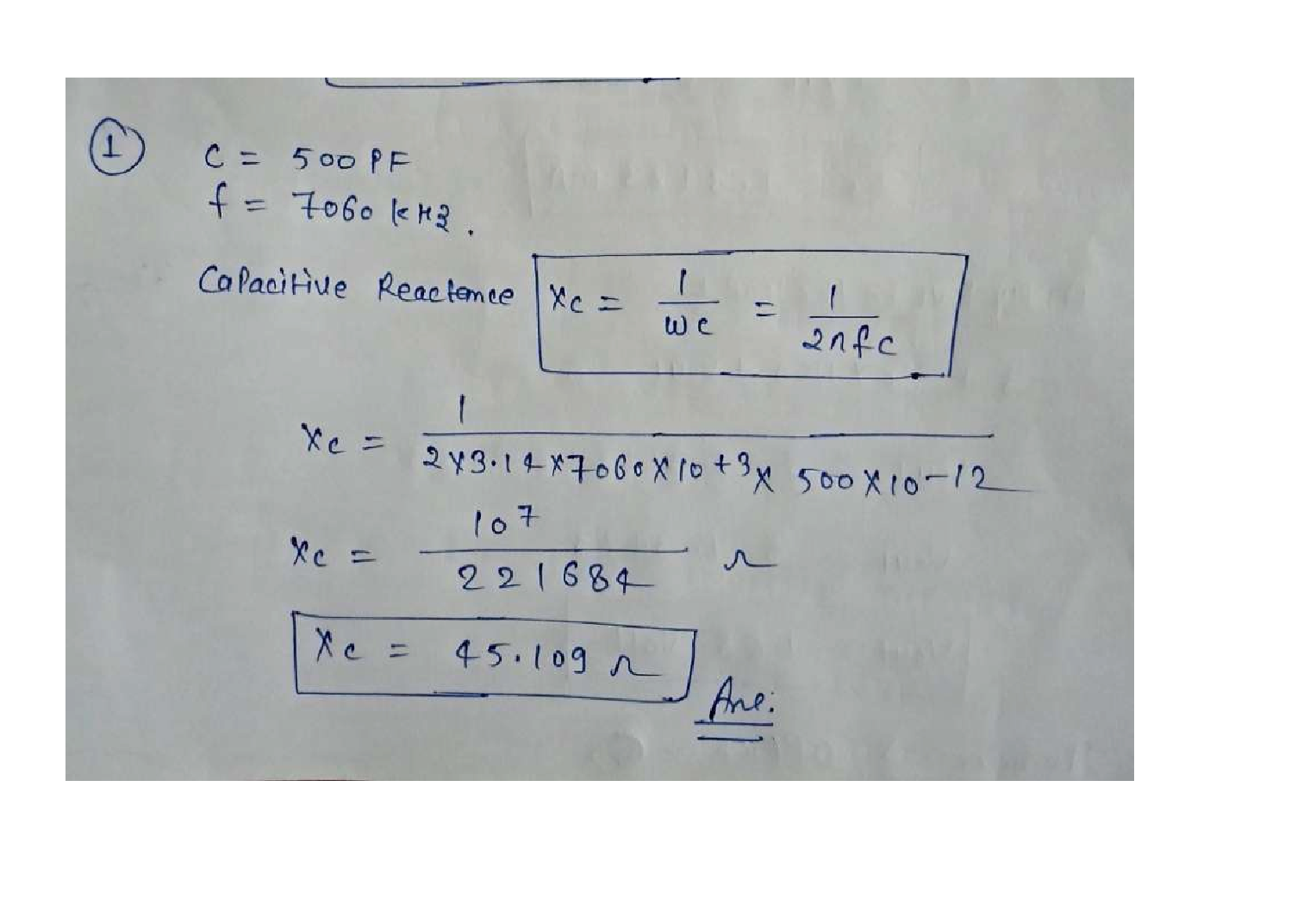Question

Circuits

What is the capacitive reactance of a 500 pF capacitor at a frequency of 7060kHz.(2)Verified### Question 45529Circuits

11) From your answer in (10), does the 4V 'DC' or steady state voltage impact the value of the displacement current, Ic.

### Question 45528Circuits

\text { 10) Assuming } I_{C}=C \frac{d V_{C}}{d t} \text {, find the displacement current for } V_{C}=e^{-\omega t}+4 V \text { and } V_{C}=
sin(wt) + 4 V.

### Question 45527Circuits

9) Based on your answer in (7), can you apply an arbitrarily large field or voltage across a dielectric? What is the name for this limitation and what is its value for Air and Glass (SiO2)?

### Question 45526Circuits

8) Remembering quantum tunneling from Chapter 3, what is happening to the probability of a tunneling event as the "thickness" of the barrier representing the dielectric decreases?

### Question 45525Circuits

7) What happens to the relative "thickness", or distance an electron must travel in a straight line across the dielectric, as you increase the voltage?

### Question 45524Circuits

6) Redraw the diagram in (5) with a small positive voltage on the right-hand side (no voltage or OVon the left-hand side).

### Question 45523Circuits

5) Draw the energy band diagram of a capacitor made of two aluminum plates with a glass dielectric. The capacitor should have zero applied voltage.

### Question 45522Circuits

\text { Given } C=\frac{\varepsilon A}{d}=\frac{q}{v} \text { and the units of } \varepsilon \text { are } F / m \text {, determine the equivalent units of the Farad (I.E. in }
terms of other available units).

### Question 45521Circuits

3) What is the result of increasing thickness of a dielectric (increasing d in the capacitor equation)while keeping the amount of charge on each plate the same? Think in terms of the capacitance and the voltage measured across the capacitor.

### Question 45520Circuits

2) Define the displacement current. Are charges physically flowing through the dielectric?

### Submit query